Get instant live expert help with Excel or Google Sheets“My Excelchat expert helped me in less than 20 minutes, saving me what would have been 5 hours of work!”

#### Post your problem and you'll get expert help in seconds

Your message must be at least 40 characters
Our professional experts are available now. Your privacy is guaranteed.

# Excel SECOND Function

While working with Excel, we are able to determine the value for seconds of any given time by using the SECOND function.  This step by step tutorial will assist all levels of Excel users in the usage and syntax of the SECOND function.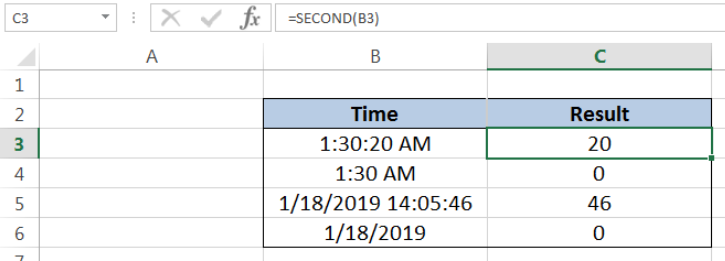Figure 1. Final result: Excel SECOND function

## Syntax of the SECOND Function

SECOND returns an integer that represents the value for seconds of any given time; value ranges from 0 to 59

`=SECOND(serial_number)`

• serial_number  – the time whose seconds value we want to find; time may be entered as decimal numbers, text strings within quotation marks, formulas or cell reference to a value of time
• The argument serial_number may also be a value representing a combination of date and time; time values are represented by a decimal number corresponding to its portion relative to one day (for example, 6:00 AM is represented as 0.25, since it is 1/4 of a day)

## Setting up our Data

Our data consists of two columns: Time (column B) and Result (column C).  We want to extract the seconds component of the time in column B. The results will be recorded in column C.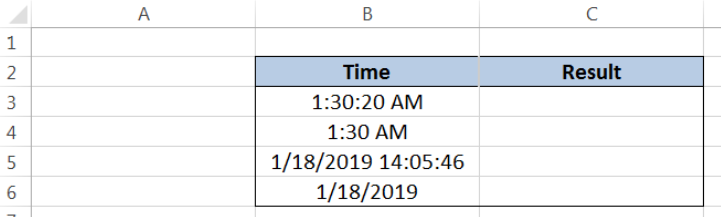Figure 2. Sample data to extract the seconds component of time

## Extract the seconds component of time

In order to determine the value for seconds of any given time by using the SECOND function, let us follow these steps:

Step 1.  Select cell C3

Step 2.  Enter the formula: `=SECOND(B3)`

Step 3.  Press ENTER

As a result, the value in cell C3 is “20”, because the seconds component of the time 1:30:20 AM in B3 is “20”.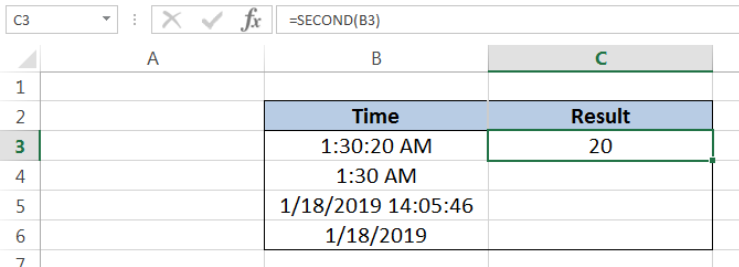Figure 3.  Using SECOND function to extract the seconds component of time

In the same way, when we enter a different time as the argument in our SECOND function, we will obtain the seconds component only.  Let us take another example:

Step 1.  Select cell C4

Step 2.  Enter the formula: `=SECOND(B4)`

Step 3.  Press ENTER

The time in B4 is 1:30:00 AM, although it is in the format hh:mm AM.  The result in cell C4 is zero “0”, because the seconds component of  1:30 AM in B4 is  “0”.Figure 4.  SECOND function extracting the seconds component of time in hh:mm format

## Extract the seconds component given a date and time

The SECOND function is able to extract the seconds component of time, even when the argument is a combination of date and time. Let us follow these steps:

Step 1.  Select cell C5

Step 2.  Enter the formula: `=SECOND(B5)`

Step 3.  Press ENTER

SECOND function completely disregards other components and returns only the value for seconds.  As a result, the value in cell C5 is “46”, from the given time of 14:05:46.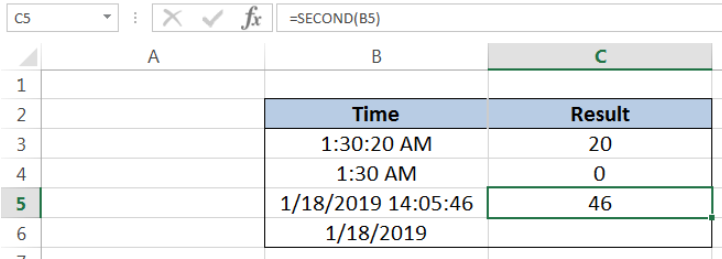Figure 5.  Output: SECOND function extracting the seconds component of time

## Notes:

• The extracted value returned by the SECOND function can be used as input to other functions such as the TIME function
• In cases where only the date is given, and there’s no time, the SECOND function returns the value zero “0”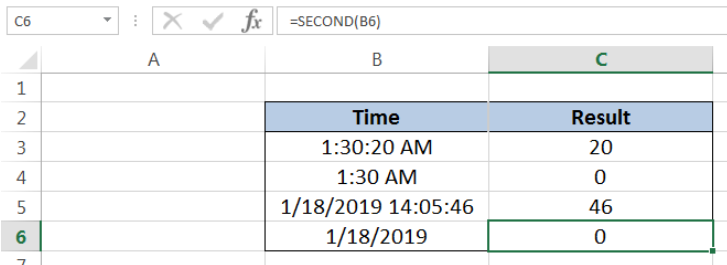Figure 6.  Output: SECOND function returns “0” if there’s no time in argument

Most of the time, the problem you will need to solve will be more complex than a simple application of a formula or function. If you want to save hours of research and frustration, try our live Excelchat service! Our Excel Experts are available 24/7 to answer any Excel question you may have. We guarantee a connection within 30 seconds and a customized solution within 20 minutes.

### Did this post not answer your question? Get a solution from connecting with the expert.Another blog reader asked this question today on Excelchat:## Subscribe to Excelchat.coAnother blog reader asked this question today on Excelchat: